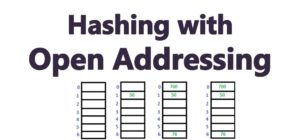Today we are going to examine Open Addressing. Recall that the two methods of resolving collisions in a hash table are:

We would cover the following:##### 1. Introduction to Hashing with Open Addressing

In Hashing with Open Addressing, all the element are placed in the hash table. Unlike Hashing With Chaining, no external linked list is used. When an element is searched for, we examine the hash table slots one after another until we find the search element or determine that the element is not in the hash table.

In open addressing, the hash table can get completely filled up with the key such that no further keys can be inserted into the table. The consequence is that  the load factor (α  = n/m) will always be at most 1.

The benefit of open-addressing over chaining is that in open addressing, pointers are avoided completely. Instead a sequence of slots to be examined (probed) is computed. Since not using pointers results in additional free memory(and more free slots), we can be sure that that there would be fewer collisions relative to when pointers are used.

##### 2. Insertion Into Hash Table With Open Addressing

To insert an element into a hash table with open addressing, we successively probe, or examine the hash table slots one after the other until an empty slot if found to insert the key. However, the sequence of slots to be probed is not sequential as this would require Θ(n) time. The sequence of slots to be examined depends on the particular key we want to insert. To determine this sequence, we use a probe number in addition to the hash function. So what we have is:

h: U x {0, 1, 2, . . . , m-1} → {0, 1, . . . , m-1}

For every key in hashing with open addressing, it is required that the probe sequence be

h(k,0), h(k,1), h(k,2), . . , h(k, m-1)

This should be a permutation of {0, 1, 2, . . . , m-1}

This means that at some point, every hash table slot is eventually examined as a position for a new key before the the table gets completely filled. The pseudocode for Hash-Insert into a hash table with open addressing is given in Listing 1.0. Here, the procedure take as input a hash table T and a key k and returns the slot number where the key is to be stored. It returns an error if the hash table is already full.

```Hash-Insert(T, k)
i = 0
do
j = h(k, i)
if T[j] == NIL
T[j] = k
return j
else
i = i + 1
while i < m
error "hash table full"
```

Listing 1.0: Pseudocode for Insert with Open Addressing

##### 3. Searching in Hash Table with Open Addressing

The insertion algorithm examines the the hash table for a key k  and follows the same probe sequence used for insertion of k. This means that if the search finds an empty slot, then key is not in the table. This is because an empty slot in the sequence means that the key should have been there, but it’s not). Also note that this is based on the assumption that keys have not being deleted from the table.

The Search procedure take a hash table T and a key k as input. It returns j if it finds a slot that contains k otherwise it returns NIL meaning that that key is not in the table.

``` Hash-Search(T, x)
i = 0
do
j = h(k, i)
if T[j] = k
return j
else
i = i + 1
while i < m and T[j] != NIL
return NIL
```

Listing 1.1: Pseudocode for Insert

##### 4. Deletion from Hash Table with Open Addressing

Deletion from hash table with open addressing is a little tricky. The reason is this: if we delete a key and mark the slot as NIL, then subsequent searches would not be able to go past this positions (since searches terminate when it finds a NIL slot). So we cannot mark a slot as NIL  when an item is deleted.

The solution to this would be to mark the slot with a special value DEL (or DELETED) instead of NIL. Then the Insert procedure would be modified to treat the the DEL slots as empty. The search procedure would then pass over the DEL slots and continue searching.

In the next lesson, we would examine the following three techniques used to compute the  probe sequence namely:

• Linear Probing